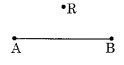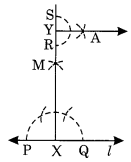# NCERT Solutions For Class 6 Maths Practical Geometry Exercise 14.4

ncert textbook

## NCERT Solutions For Class 6 Maths Practical Geometry Exercise 14.4

NCERT Solutions For Class 6 Maths Chapter 14 Practical Geometry Ex 14.4

Exercise 14.4

Ex 14.4 Class 6 Maths Question 1.
Draw any line segment$\overline { AB }$. Make any point M on it. Through M, draw a perpendicular to$\overline { AB }$. (Use ruler and Compasses)
Solution:
Step I : Draw a line segment$\overline { AB }$and mark any point M on it.
Step II : Put the pointer of the compass at M and draw an arc of suitable radius such that it intersects$\overline { AB }$at P and Q.Step III : Take P and Q as centres and radius greater than PM, draw two arcs such that they intersect each other at C.
Step IV : Join M and C.
Thus CM is the perpendicular to$\overline { AB }$.

Ex 14.4 Class 6 Maths Question 2.
Draw any line segment$\overline { PQ }$. Take any point R not on it. Through R, draw a perpendicular to$\overline { PQ }$. (Use ruler and set square).
Solution:
Step I: Draw a line segment$\overline { PQ }$and a point R outside of$\overline { PQ }$.
Step II : Place a set square on$\overline { PQ }$such that one side of its right angle be along it.

Step III: Place a ruler along the longer side of the set square.Step IV : Hold the ruler fix and slide the set square along the ruler till it touches the point R.Step V : Join RM along the edge through R. Thus$\overline { RM }$$\overline { PQ }$.

Ex 14.4 Class 6 Maths Question 3.
Draw a line l and a point X on it. Through X, draw a line segment$\overline { XY }$perpendicular to l. Now draw a perpendicular to$\overline { XY }$at y. (Use ruler and compasses)
Solution:
Step I: Draw a line l and take a point X on it.
Step II : Draw an arc with centre X and of suitable radius to intersect the line l at two points P and Q.Step III : With P and Q as centres and a radius greater than P draw two arcs to intersect each other at M.
Step IV : Join XM and produce to Y.
Step V : With Y as centre and a suitable radius, draw an arc to intersect XY at two points R and S.
Step VI: With R and S as centres and a radius greater than YR, draw two arcs to intersect each other at A.
Step VII: Join Y and A. Thus YA ⊥ XY.